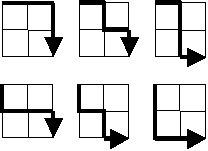# Java solution to Project Euler Problem 15

Starting in the top left corner of a 22 grid, there are 6 routes (without backtracking) to the bottom right corner.How many routes are there through a 2020 grid?

Running time: 0ms

Assessment: I was totally lost on this problem, and I eventually had to search for some clue as to how to solve it. Once I learned it was an n-choose-k problem, it was a lot easier. I used the simplified binomial coefficient algorithm described in the Wikipedia article, but only after making sure I understood what was going on and why it was used.

This simplified method ensures you don’t overflow your primitives when calculating factorials, and it’s ridiculously fast. (As I recall, it’s much faster than the ideal solution posted by the project admins.)

In retrospect, it seems obvious that it’s a combinatorics problem, because you can’t go backwards or up, but that wasn’t apparent to me when I started.

```public class Problem015
{
public static long binomialCoefficient(int n, int k)
{
/* N-choose-k combinatorics: (n! / (k! * (n-k)!)
* Where:
* 		n is the number of moves,
* 		k is the number of down and right moves required (20 each) */

if (k > (n-k))
k = n - k;

long c = 1;

for (int i = 0; i < k; i++)
{
c = c * (n-i);
c = c / (i+1);
}

return c;
}

public static void main (String[] args)
{
long begin = System.currentTimeMillis();

System.out.println(binomialCoefficient(40,20));

long end = System.currentTimeMillis();
System.out.println(end-begin + "ms");
}
}```

## 1 thought on “Java solution to Project Euler Problem 15”

1.Chandan says:

Hi ,

For 22 grid , u r answer wont give 6 as the answer.
For 32 grid also the solution , is bad.

How does this problem became the Combinatorics problem , please explain.